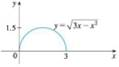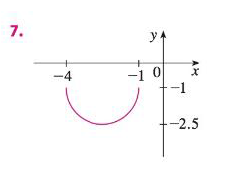Chapter 1.3, Problem 7E### Single Variable Calculus: Early Tr...

8th Edition
James Stewart
ISBN: 9781305270343

#### Solutions

Chapter
Section### Single Variable Calculus: Early Tr...

8th Edition
James Stewart
ISBN: 9781305270343
Textbook Problem

# The graph of y = 3 x − x 2 is given. Use transformations to create a function whose graph is as shown.To determine

To create: A function from the given graph by using transformations.

Explanation

The equation of the given graph is y=3xx2 .

Let y=f(x) .

Notice that the given graph is a semi-circle with the domain [0, 3] and the range [0, 1.5].

Also, it is observed from the given graph that the graph is shifted 4 units to the left and shifted 1 unit downward.

Moreover, the graph is reflected about the origin.

Thus, add 4 to x as it shifted 4 units to the left; subtract 1fromf(x)as it shifted 1 unit downward and then multiply f(x) by −1 as the graph reflects about x-axis

### Still sussing out bartleby?

Check out a sample textbook solution.

See a sample solution

#### The Solution to Your Study Problems

Bartleby provides explanations to thousands of textbook problems written by our experts, many with advanced degrees!

Get Started

#### Find an equation of the line that passes through (1, 2) and (4, 5).

Applied Calculus for the Managerial, Life, and Social Sciences: A Brief Approach

#### In Problems 7-34, perform the indicated operations and simplify. 12.

Mathematical Applications for the Management, Life, and Social Sciences

#### Evaluate the integral. 26. 013x2+1x3+x2+x+1dx

Single Variable Calculus: Early Transcendentals

#### The length of the curve given by x = 3t2 + 2, y = 2t3, is:

Study Guide for Stewart's Single Variable Calculus: Early Transcendentals, 8th

#### Write a research report using APA style.

Research Methods for the Behavioral Sciences (MindTap Course List)